Symmetry Restraint for Solid Models

For solid models, every face that is coincident with a plane of symmetry should be prevented from moving in its normal direction. The Symmetry restraint type applies this condition automatically to all selected faces. The planes of symmetry should be orthogonal to each other.

Symmetry requires that geometry, restraints, loads, and material properties are symmetrical. In general, using symmetry is not recommended for buckling and frequency studies.

The following are some examples of models that are symmetric with respect to one or more planes.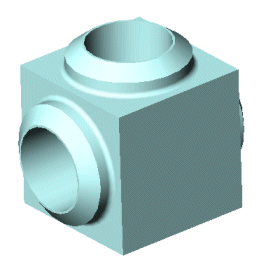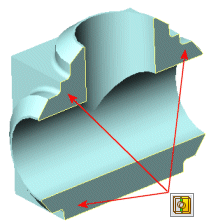This model is symmetrical with respect to one plane. You can analyze half of the model. Apply the Symmetry restraint type to the faces of symmetry.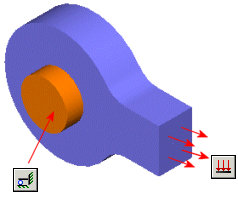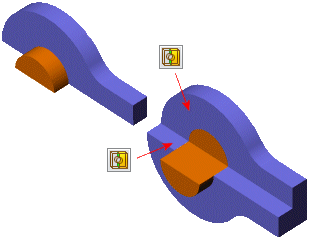This model is symmetrical with respect to two planes. You can analyze one quarter of the model. Apply the Symmetry restraint type to the faces of symmetry. In case the model is symmetric about three planes, you can analyze one eighth of the model.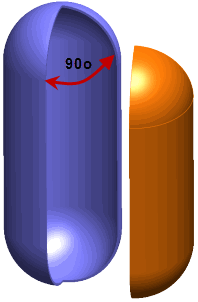This model is axisymmetric. If the loads are such that the cut sections cannot deform normal to their planes (for example pressure loads that act radially), you can analyze a wedge. Use an angle for the wedge that is divisible by 360o. Practically, you should not use a very small angle that can result in a bad mesh. If tangential loads exist and the cut sections can deform normal to their planes, use cyclic symmetry.